Get instant live expert help with Excel or Google Sheets“My Excelchat expert helped me in less than 20 minutes, saving me what would have been 5 hours of work!”

#### Post your problem and you’ll get Expert help in seconds.

Your message must be at least 40 characters
Our professional Expert are available now. Your privacy is guaranteed.

# How to Search Multiple Worksheets for Value

We can search MULTIPLE WORKSHEETS for a certain value and get a numeric count for the number of occurrence of the value with a formula that integrates the COUNTIF and the INDIRECT function. The steps below will walk through the process.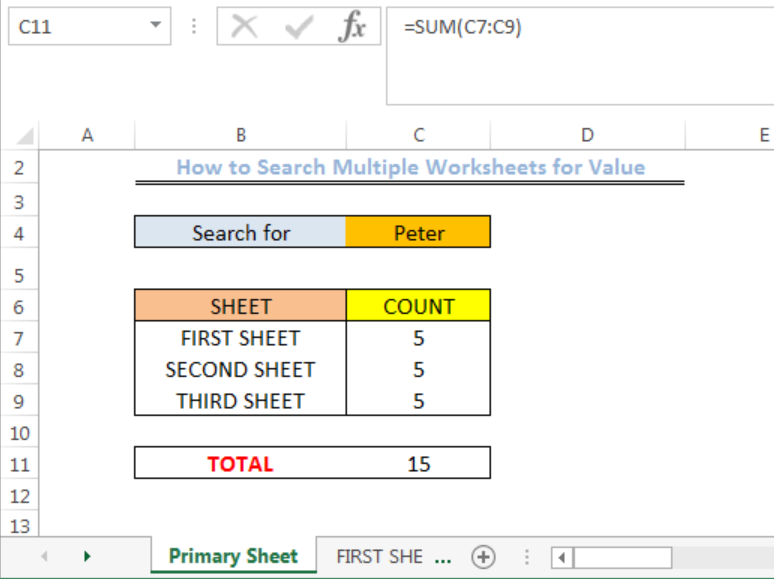Figure 1: How to Search Multiple Worksheets for Value

## Formula

The formula in Cell C7 is:

`=COUNTIF(INDIRECT("'"&B7&"'!"&"1:1048576"),\$C\$4)`

## Setting up the Data

We will set up the data to search for Peter and get a numeric count by doing the following:

• We will open an EXCEL WORKSHEET
• We will type the data into the PRIMARY SHEET, FIRST SHEET, SECOND SHEET, and THIRD SHEET. On the FIRST SHEET, SECOND SHEET, and THIRD SHEET, we are searching for the number of times the name PETER appears.
• Note: We have similar data in the FIRST SHEET, SECOND SHEET, and THIRD SHEET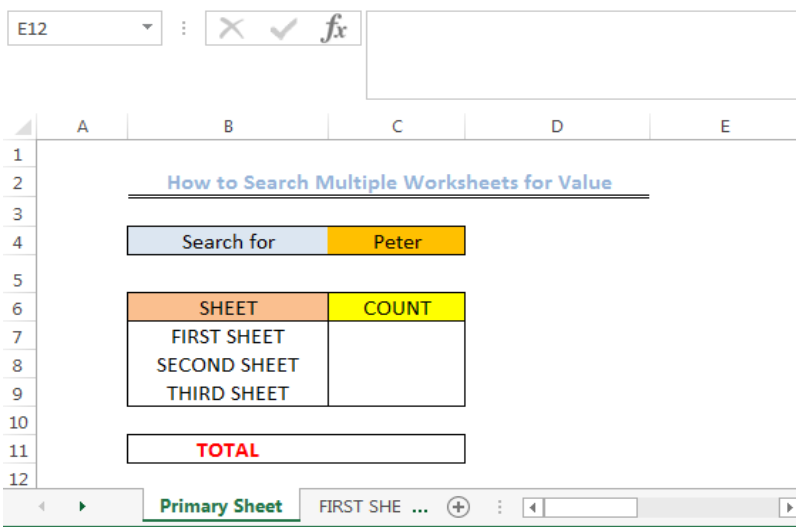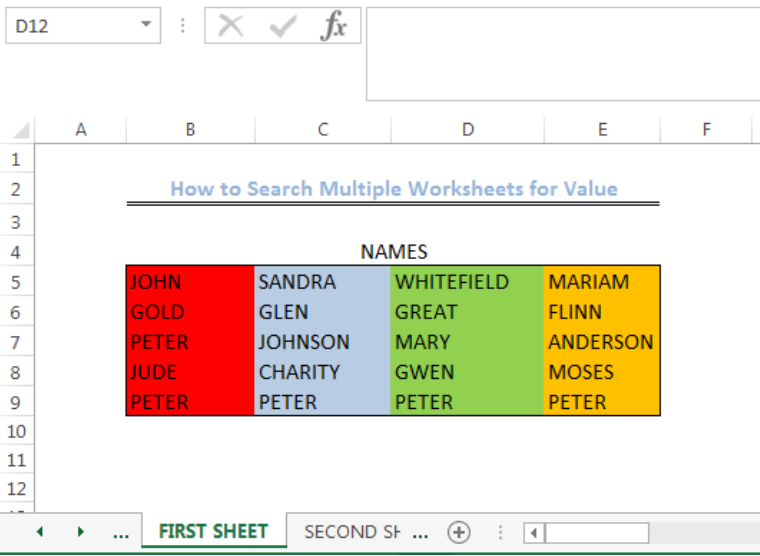Figure 2: Setting up the Data

## Search Multiple Worksheets for Value

To search the first, second and third sheet for the name PETER, we will do the following:

• We will click on Cell C7 in the PRIMARY SHEET

• We will insert the formula below into Cell C7

`=COUNTIF(INDIRECT("'"&B7&"'!"&"1:1048576"),\$C\$4)`

• We will press the enter key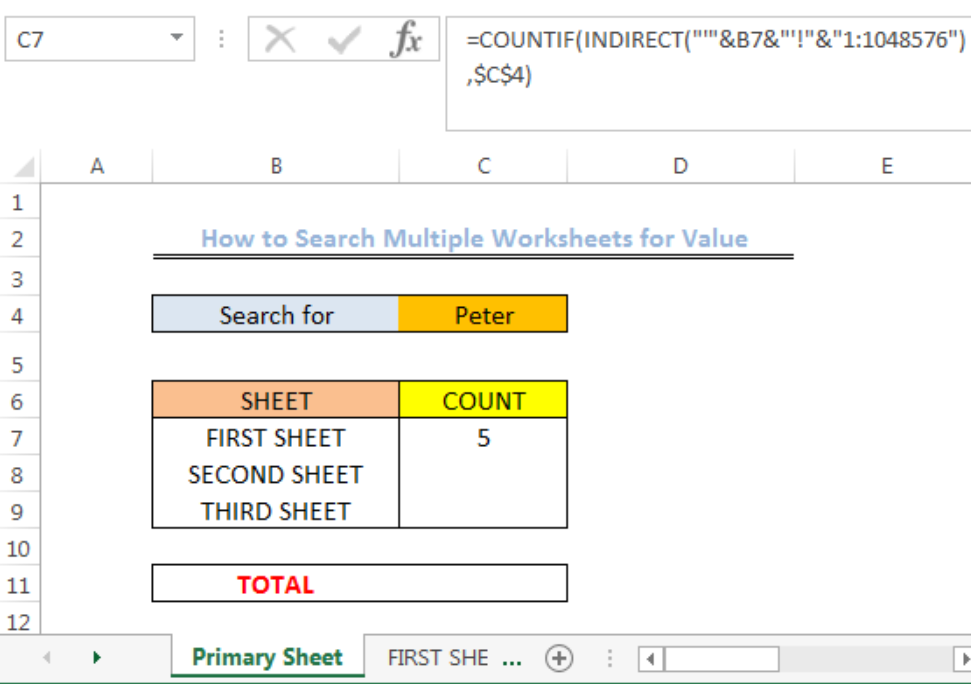Figure 3: Search and Count for PETER in the FIRST SHEET

• We will use the fill handle to drag down and copy the formula into Cells C8 and C9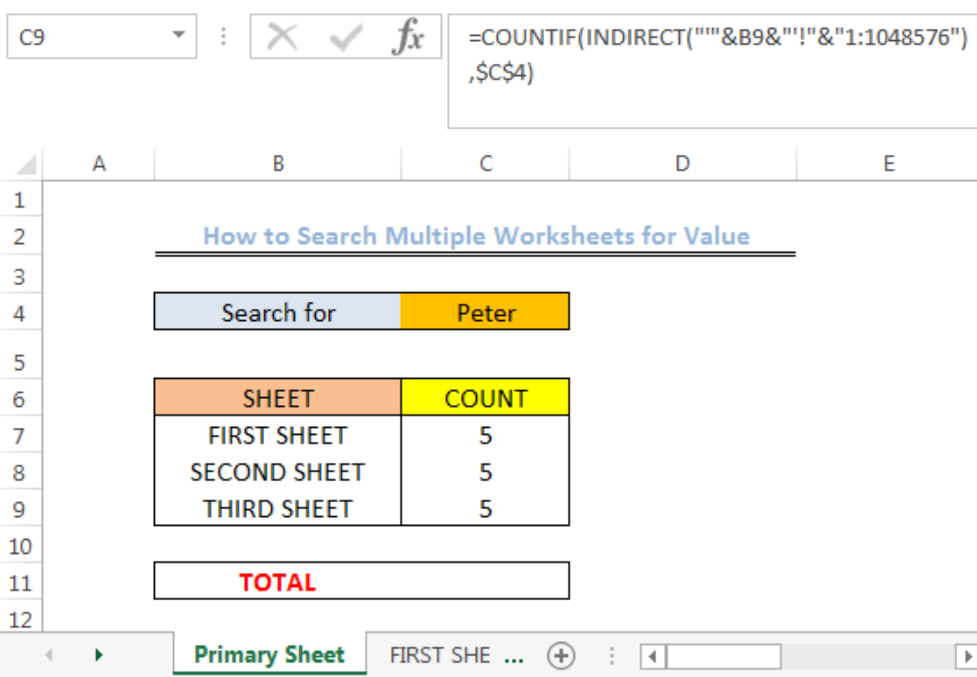Figure 4: Search and Count for PETER in the FIRST, SECOND and THIRD SHEET

• We will sum the count by clicking on Cell C11 and input the formula below

`=SUM(C7:C9)`

• We will click the ENTER button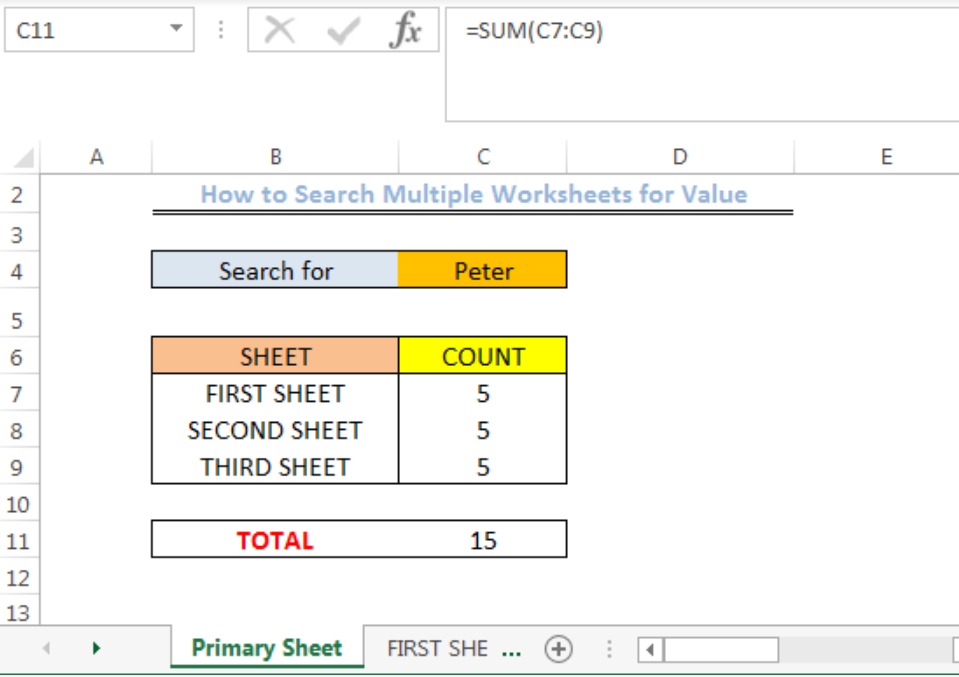Figure 5: Sum of the Number of Occurrences of PETER in the FIRST, SECOND and THIRD SHEET

## Explanation

The INDIRECT function returns as a text the value in Cell C4 of the Primary Sheet. The COUNTIF function counts the number of cells that the text appears in as returned by the INDIRECT function and returns the value which is 5 for each sheet. The SUM function totals the count of the range C7:C9 to give 15.

## Instant Connection to an Expert through our Excelchat Service

Most of the time, the problem you will need to solve will be more complex than a simple application of a formula or function. If you want to save hours of research and frustration, try our live Excelchat service! Our Excel Experts are available 24/7 to answer any Excel question you may have. We guarantee a connection within 30 seconds and a customized solution within 20 minutes.

### Did this post not answer your question? Get a solution from connecting with the expert.Another blog reader asked this question today on Excelchat:
Solution examplesHello, I need help regarding indirect formula of excel. 1. What is the purpose of using this formula? 2. If we use this formula with sum formula, then why we are using this with sum formula, we can also perform addition from different sheets without indirect formula ?
Solved by O. D. in 21 minsI need a formula to pop at the upper left corner of a spreadsheet. If I enter the month "January," I want the column number sum of January =SUM(AB11:AB75) from another section on the same excel page to pop right below the "January" cell, and not display the formula expression, but see the \$100.
Solved by T. Q. in 40 minsCan't add (SUM) in imported numbers from bank account
Solved by F. C. in 40 minsI need a formula to combine D2 to D100 to add together a column of numbers, then take away the same amount on the same row when column E is filled. i.e. column D is a price of an item, so the formula must calculate the total, then when the item is sold an 'a' is marked next to the item in column E, the formula then must deduct this amount from the total
Solved by X. W. in 20 minsI would like to have a diagram in a new sheet, where the horizontal axis is the days, as they are in column DX. Each day shall show the sum of all unique leads of that day, and I would like to be able to check via a box of checkboxes, which facilities are shown, the facilities are in column BC.
Solved by I. A. in 45 mins## Subscribe to Excelchat.coAnother blog reader asked this question today on Excelchat: